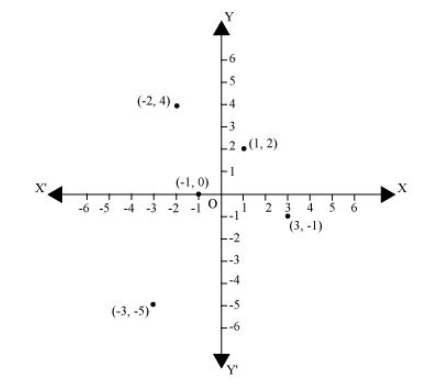# In which quadrant or on which axis do each of the points $(-2,4),(3,-1),(-1,0),(1,2)$ and $(-3,-5)$ lie? Verify your answer by locating them on the Cartesian plane.Solution:The point $(-2,4)$ lies in the $I I^{\text {nd }}$ quadrant in the Cartesian plane because for point $(-2,4), x$-coordinate is negative and $y$-coordinate is positive.

Again, the point $(3,-1)$ lies in the IV $^{\text {th }}$ quadrant in the Cartesian plane because for point $(3,-1), x$-coordinate is positive and $y$-coordinate is negative.

The point $(-1,0)$ lies on negative $x$-axis because for point $(-1,0)$, the value of $y$-coordinate is zero and the value of $x$-coordinate is negative.

The point $(1,2)$ lies in the $I^{\text {st }}$ quadrant as for point $(1,2)$, both $x$ and $y$ are positive.

The point $(-3,-5)$ lies in the III $^{\text {rd }}$ quadrant in the Cartesian plane because for point $(-3,-5)$, both $x$ and $y$ are negative.